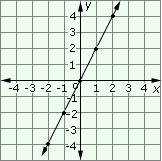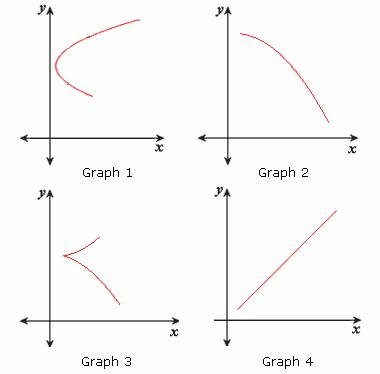## Definition Of Linear Function

A function that can be graphically represented in the Cartesian coordinate plane by a straight line is called a Linear Function.

A linear function is a first degree polynomial of the form, F(x) = m x + c, where m and c are constants and x is a real variable.
The constant m is called slope and c is called y-intercept.

### Example of Linear Function

y = 3x + 5 is a linear function.
The graph of the function y = 2x is shown below. This is a linear function since the points fit onto a straight line### Solved Example on Linear Function

#### Ques: Identify the graph that represents a linear function.A. Graph 1
B. Graph 3
C. Graph 4
D. Graph 2